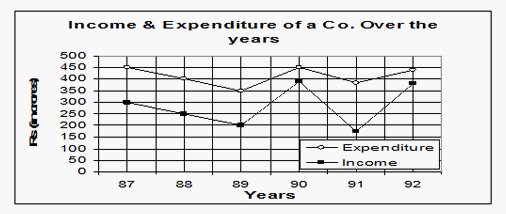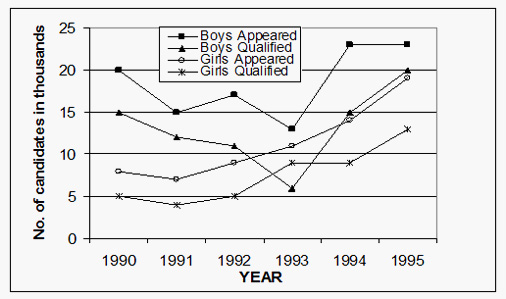# Line Graph Practice Questions: Level 01

DIRECTIONS for questions 1-5: Study the following graph carefully and answer the line graph problems given below:1. The aggregate expenditure of which of the following sets of years was equivalent to the income in 1990?
A. 1987 & 1988
B. 1987 & 1989
C. 1988 & 1989
D. None of these
2. What was the approximate percentage decrease in income from 1987 to 1988?
A. 80%
B. 50%
C. 17%
D. 40%
3. In what number of the given years was the expenditure more than the average expenditure of the given years?
A. 4
B. 3
C. 1
D. 6
4. In which of the following years was the ratio of expenditure to the income, the lowest?
A. 1987
B. 1988
C. 1989
D. 1990
5. What was the approximate percentage increase in expenditure from 1989 to 1990?
A. 35%
B. 40%
C. 20%
D. None of these
DIRECTIONS for questions 6-10: Study the following line chart carefully and answer the questions given below:
Number of candidates (Girls & Boys) Appeared & Qualified Over the Years6. The percentage (approximate) of boys qualified to appear in the year 1995 was?
A. 35%
B. 86.95%
C. 80%
D. 65%
7. Which two consecutive years had the same number of qualified girls?
A. 1994 and 1995
B. 1993 and 1994
C. 1991 and 1992
D. 1992 and 1993
8. What was the % increase in the no. of boys qualified from 1994 to 1995?
A. 50%
B. 33.33%
C. 100%
D. 150%
9. The difference between the total number of boys & girls qualified in 1990 and 1995 was
A. 5000
B. 2000
C. 7500
D. 13000
10. Which year had the lowest percentage of girls qualified to appeared among the years given?
A. 1991
B. 1993
C. 1994
D. 1992